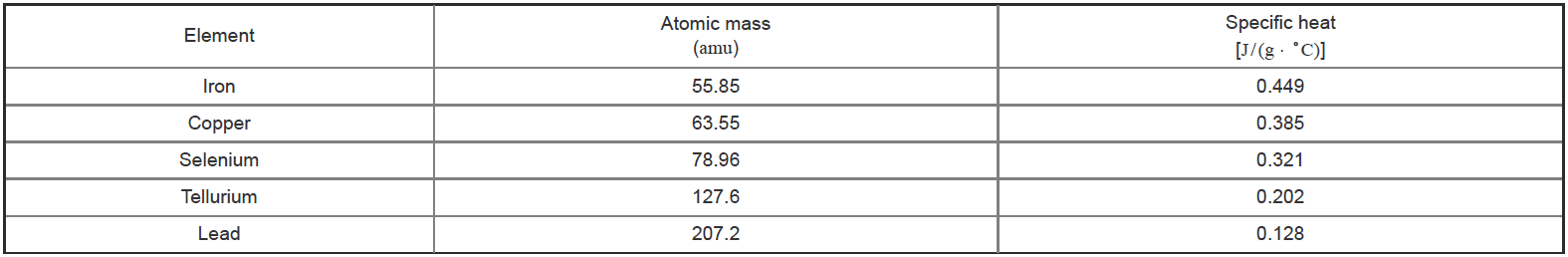# Problem: A linear plot refers to a straight line created when two variables are graphed. Use the data displayed in the table to determine which of the following plots will result in a linear graph relating specific heat and atomic mass.Check all that apply.__ specific heat versus atomic mass__ specific heat versus (atomic mass)−1__ specific heat versus √atomic mass__ specific heat versus (atomic mass)2Based on specific heat measurements, Pierre Dulong and Alexis Petit proposed in 1818 that the specific heat of an element is related to its atomic weight (atomic mass). Thus, by measuring the specific heat of a new element, its atomic weight could be readily established. The specific heat and atomic mass for a series of elements are provided for reference.

###### FREE Expert Solution
99% (103 ratings)View Complete Written Solution
###### Problem Details

A linear plot refers to a straight line created when two variables are graphed. Use the data displayed in the table to determine which of the following plots will result in a linear graph relating specific heat and atomic mass.

Check all that apply.

__ specific heat versus atomic mass
__ specific heat versus (atomic mass)−1
__ specific heat versus √atomic mass
__ specific heat versus (atomic mass)2

Based on specific heat measurements, Pierre Dulong and Alexis Petit proposed in 1818 that the specific heat of an element is related to its atomic weight (atomic mass). Thus, by measuring the specific heat of a new element, its atomic weight could be readily established. The specific heat and atomic mass for a series of elements are provided for reference.